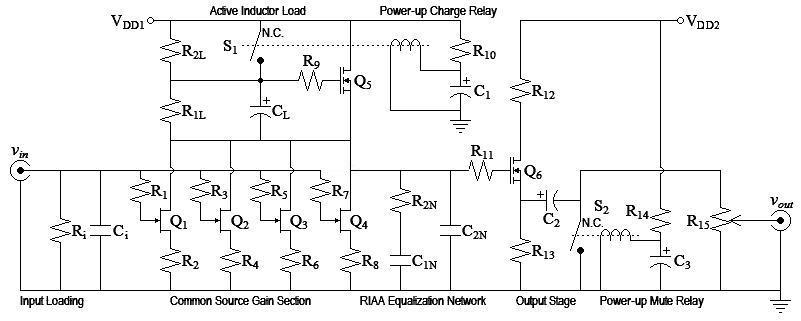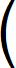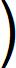Home  │ Audio Home Page

Updated September 2, 2010.  See document history at end for details.

## Low-Noise JFET Phono Preamp

This is an example only.  It is not intended to be final or complete design.  It should only serve as an example of equalization and active inductor calculations and not be built.  The SPICE verified two stage design Low Noise 2-Stage All JFET Phono Preamp would better serve building an actual prototype.
This is a redesign using LSK170 JFETs on the input.  You still may want to use the 2N3819 design because of possible difficulty of obtaining LSK170's.

Figure 1:  SchematicParts List Q1-Q4 LSK170C n-ch jfet Q5,Q6 BS170 n-ch mosfet R1,R3,R5,R7 56Ω, 5% R2,R4,R6,R8 56Ω, 5% R2L 56.2kΩ, 1% R2N 8.25kΩ, 1% C1N,a 39nF, 1% or 5% C1N,b unneeded C2N,a 13nF, 1% or 5%
 C2N,b 360pF, 1% or 5% R1L 38.3kΩ, 1% CL 10,000µF, 25V electrolytic R9,R11 1kΩ, 5% R12 82Ω, 5% R13 1kΩ, 5%  (or 220Ω, see text) C2 100µF, 25V electrolytic R15 1kΩ pot (or 2kΩ, see text) S1,S2 SPST reed relay, normally closed, coil rated 10mA@5V
Remaining values are left for you to calculate.  See text.

#### Initial Design Decisions

• Discrete voltage input design with 46db gain at 1kHz will amplify 5mV rms input to produce 1V rms before attenuator.
• Q1-Q4 are LSK170 n-ch jfet (chosen for excellent 1nV/rtHz noise specification).  Use C grade to accommadate current requirements.
• I chose four devices because multiple parallel devices devices increase S/N by sqrt(# of devices).  In this case bettering the effective noise specification to 0.866nV/rtHz.  (Gate and source resistor choices prevent ideal effective input noise of 0.5nV/rtHz)
• Q5,Q6 are BS170 n-ch mosfet (commonly available)
• vgs,max of Q6 of 20V limits VDD due to startup relays.  Choose VDD = 18V.
• Let bias of active inductor set bias to gate of Q6 to eliminate coupling capacitor.
 vg,Q6 = (VDD-vgs-Q5-max)+vsg-Q1-max 2 = 18V-2V+8V 2 = 12V
• R2L of active inductor functions as R1 of passive RIAA network.
• I chose to do the small signal class-a design with a fourier series based distortion predictor tool out of convenience.  Small signal class-a design is not in the scope of this article.
• Because of possibility of negative feedback from buffer stage back to gain stage through power supply, I decided to recommend two power supply taps.

#### Gain Stage Design

Although I used a convenient fourier series analysis tool to do the small signal analysis, there were some design decisions.  I wanted to choose gate and source resistors small enough to make their noise contribution insignificant compared to the JFET.  This would lower noise and allow the possiblity of noise improvement with better JFETs.
 Resistor noise equation (per rtHz, figure of merit, does not account for bandwidth) vn-resistor-rtHz = sqrt(4kTR)
Rearrange resistor noise equation to calculate equivalent input noise resistance of JFET.
 R = vn-resistor-rtHz2 4kT = 1nV2 4 x 1.3806504e-23 x 298.15ºK = 60.73254378Ω
I would like to choose RG and RS much lower than 60Ω to make their contribution insignificant.  However, I believe a 6Ω source resistor would greatly raise distortion and bias for a small signal transistor.  Rather, instead I will match the source resistor to the JFET noise resistance.
R1-R8 = 56Ω.
Because the fourier series analysis of this low noise design showed higher distortion than the 2N3819 version (but still very good) and because decreasing the source resistors furthur would increase the distortion, I made no furthur analysis with lower source resistor values.

#### Fourier Series Analysis of Q1-Q4 (excluding drain load)

Analysis of Common Source Circuit
transistor type = JFET (Depletion mode FET approximation)
IDSS = 12.25mA
MOSFET constant = 25mS
threshold voltage = -700mV
source resistor = 56Ω

Input signal (peak) = 7.071mV
Input bias = 0V
Output signal (peak) = 69.341565µA
Output bias = 4.7314585mA
Gain = 9.8064722mS

Total distortion = 57.385979nA = 0.08275841% = -61.6438dB

Breakdown by harmonic

 harmonic value percent dB 0 4.7315158mA 6823.49150778% 36.68dB DC Offset 1 69.341565µA 100.00000000% 0.00dB Fundamental 2 57.270744nA 0.08259223% -61.66dB 3 115.23468pA 0.00016618% -115.59dB

Distortion figures for first stage are before RIAA equalization.  At the output of the RIAA network, they are lower because of the downward sloping equalization. (A half or a little more for frequencies on or beginning the downward slope; in decibels, 6db lower)

#### RIAA Analysis

Refer Calculating Passive RIAA Equalization in article Phono Equalization Calculations.

From this fourier analysis we can calculate R1 of Passive RIAA network (aka R2L)
 R2L = (Low frequency RIAA boost relative to 1kHz) x (1kHz output voltage) (Output signal current) x (#parallel devices) = 10 x 1.414213562V 69.341565µA x 4 = 56.08594638kΩ
Round to nearest 1% value.  R2L = 56.2kΩ

Given R1:R2 = 6.877358491
 R2N = 56.2kΩ 6.877358491 = 8.171742112kΩ
Round to nearest 1% value.  R2N = 8.25kΩ

Given R1C1 = 2187µS
 C1N = 2187µS 56.2kΩ = 38.91459075nF
Round down to nearest 5% value: 39nF
Choose parallel 5% value to makeup remainder:  0
C1N = 39nF + 0

Given R1C2 = 750µS
 C2N = 750µS 56.2kΩ = 13.34519573nF
Round down to nearest 5% value: 13nF
Choose parallel 5% value to makeup remainder:  360pF
C2N = 13nF + 360pF

#### Active Inductor Analysis

• Choose R2L for gain of overall circuit.
R2L chosen already in RIAA analysis.
BS170 specification:  gfs = 320mS @ 200mA.

Calculate MOSFET constant from gfs@iD  specification.
 kn = gfs2 4iD = 320mS2 4(200mA) = 128mA/V2

Calculate new gfs from operating current and  kn.

iD = 4.7314585mA x 4 - (4V/56.2kΩ)  =   18.925834mA - 71.42857143µA = 18.85440543mA
gfs = 2 x sqrt(kniD) = 2 x sqrt(128mA/V2 x 18.85440543mA) = 98.25200038mS

R1L =

R2LVbias vT+sqrt(iD/kn) -1=
56.2kΩ6V 2V+sqrt(18.85440543mA/98.25200038mS) -1= 38.46756952kΩ
Round to nearest 1% value:  R1L = 38.3kΩ
• Choose CL to set the pole frequency the desired amount below the passband.  (equation (21))
 CL = gfsR1L+1 2πfPOLER1L = (98.25200038mS x 38.3kΩ)+1 2π x 5Hz x 38.3kΩ = 3.127458306mF
3300µF would work here.  I would rather choose a larger common value (CL = 10,000µF) and solve for a lower pole.
 fPOLE = gfsR1L+1 2πCLR1L = (98.25200038mS x 38.3kΩ)+1 2π x 10mF x 38.3kΩ = 1.563729153Hz
• Determine if a bypass switch is needed to charge the capacitor on startup.
Power on relay added at beginning of design.
Calculate charge time for CL:
 i = C δv δt
Rearrange capacitor equation above and calculate time.  (2V presumes vgs not much more than vT)
 Δt = CΔv iD = 10mF x 2V 18.85440543mA = 1.060760048Sec

#### Q6 Circuit Design

My design goal here was reasonably low output impedance.  1kΩ output impedance would have been low enough to minimize voltage loss into a typical 47kΩ load.  I choose 500Ω instead on the far chance of driving a 600Ω load with a tolerable voltage loss.

#### Fourier Series Analysis of Q6 Circuit

Note:  Bias and source resistor represent equivalent circuit simplified for analysis.

Original Design R13 = 1kΩ, R15 = 1kΩ.  Large 2nd harmonic dominates distortion.  Expect to be very tubey

Analysis of Common Source Circuit
transistor type = MOSFET
MOSFET constant = 128mS
threshold voltage = 2V
source resistor = 500Ω

Input signal (peak) = 1.4142136V
Input bias = 7V
Output signal (peak) = 2.7487914mA
Output bias = 9.4563897mA
Gain = 1.943689mS

Total distortion = 3.0913658µA = 0.11246273% = -58.9798dB

Breakdown by harmonic

 harmonic value percent dB 0 9.4592357mA 344.12344914% 10.73dB DC Offset 1 2.7487914mA 100.00000000% 0.00dB Fundamental 2 2.8643766µA 0.10420495% -59.64dB 3 206.32369nA 0.00750598% -82.49dB 4 18.586854nA 0.00067618% -103.40dB 5 1.875836nA 0.00006824% -123.32dB 6 202.86746pA 0.00000738% -142.64dB

For a somewhat more neutral sound, consider that raising the bias relative to the signal will lower the distortion.
Lower R13 and raise R15 to do this.
Alternative design:  R13 = 220Ω, R15 = 2kΩ.

Analysis of Common Source Circuit
transistor type = MOSFET
MOSFET constant = 128mS
threshold voltage = 2V
source resistor = 200Ω

Input signal (peak) = 1.4142136V
Input bias = 11V
Output signal (peak) = 6.8377279mA
Output bias = 42.131413mA
Gain = 4.8350036mS

Total distortion = 4.7943711µA = 0.07011644% = -63.0836dB

Breakdown by harmonic

 harmonic value percent dB 0 42.136007mA 616.22819497% 15.79dB DC Offset 1 6.8377279mA 100.00000000% 0.00dB Fundamental 2 4.6032189µA 0.06732088% -63.44dB 3 181.69099nA 0.00265718% -91.51dB 4 8.965676nA 0.00013112% -117.65dB 5 495.5468pA 0.00000725% -142.80dB

#### Miscellaneous Calculations

R12 limits charge current through Q6 to C2 to <500mA
R12 >= 20V/500mA = 40Ω
Choose R12 = 82Ω.

Want to set C2 for as low a highpass pole as CL.  First find Requiv of pole.  Output impedance of Q6 is the inverse of its operating ac transconductance gfs.
gfs = 2 x sqrt(kniD) therefore
 Rout-Q6 = 1 2 x sqrt(kniD) = 1 2 x sqrt(128mA/V2 x 42.131413mA) = 6.808665637Ω

More tubey version:
 Requiv = (Rout-Q6 || R13) + R15 = Rout-Q6 x R13 Rout-Q6 + R13 + R15 = 1.006762621kΩ

 C2 = 1 2πfpoleRequiv = 1 2π x 2.245671674Hz x 1.006762621kΩ = 70.39580633µF
Choose C2 = 100µF.

The same calculations for the more neutral version give
Requiv = 2.006604273kΩ and C2 = 35.31930408µF
Choose C2 = 47µF.

Choose R10 and  R14 to set 10mA through relay coil.
 R10 = VDD-vCOIL iCOIL = 18V-5V 10mA = 1.3kΩ

Calculate load current from power supplies VDD1 and VDD2.
iDD1 = iQ1-BIAS x 4 + iRELAYCOIL = 18.85440543mA + 10mA  = 28.85440543mA
iDD2 = iQ6-BIAS + iRELAYCOIL = 9.4563897mA + 10mA = 19.4563897mA (more tubey version)
iDD2 = iQ6-BIAS + iRELAYCOIL = 42.131413mA + 10mA = 52.131413mA (more neutral version)

#### Design Decisions Left to You

• Ri and Ci should be calculated per article Phono Termination Calculations and Calculator.
• Gate resistors were somewhat arbitrarily chosen.  They seem to be good values.  I know that the 1kΩ values work with the BS170.  I have seen many jfet schematics without gate resistors at all.  Increase them if any oscillations occur.  Because the gate resistor noise in this design is the same as that of the JFET chosen, I suggest trying to lower or eliminate gate resistors on the JFETs, so long as they do not oscillate.
• Relay components C1 and C3 must be calculated as follows:
C1 must be chosen by calculation or experimentation to exceed CL charge time calculated above (432.2244809mSec).  C3 would then be chosen for a somewhat longer delay to prevent a pop from the activation of the other relay.
• This circuit and its analysis is only given as an example; change everything if you like.
• A low noise 18V power supply.  I recommend the circuit of article Line Level Class A Power Supply.

1There is a seeming discrepancy in the fourier analysis between the cited output bias and the same value given in the analysis.  The first value is the bias without any signal and the second with a signal. This effect is created by the same asymetrical transfer curve that gives us a desireable second order distortion characteristic.  The positive half of the signal is amplified more than the negative half.  As a result the dc bias is modulated by the average signal level. This small component amounts to AM demodulation of the  music signal.  Abstractly, I had already anticipated this effect.  I would be curious what effect this has on the overall sound.  Is is detrimental, or does it add an extra euphonic bass urge?

Document History
January 2, 2010  Created